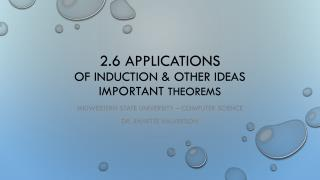DownloadDownload Presentation2.6 Applications Of Induction & other ideas Important Theorems

# 2.6 Applications Of Induction & other ideas Important Theorems

Download Presentation## 2.6 Applications Of Induction & other ideas Important Theorems

- - - - - - - - - - - - - - - - - - - - - - - - - - - E N D - - - - - - - - - - - - - - - - - - - - - - - - - - -
##### Presentation Transcript

1. 2.6 ApplicationsOf Induction & other ideasImportant Theorems Midwestern State University – Computer Science Dr. Ranette Halverson

2. Theorem 2.8 – Binary Search • For any non-negative integer n, at most n+1 comparisons are required to determine if a particular number is in a list of 2n sorted numbers. • Alternative: For any sorted list of n numbers, at most log2 n + 1 comparisons are required to determine if a particular number is in the list. • Binary Search Complexity: O (log2n)

3. Theorem 2.9 – Merge sorted lists • Let A & B be sorted lists of numbers (both decreasing or increasing). Assume positive integer n represents the combined total of numbers in the 2 lists. A & B can be merged into a single list of sorted numbers in at most n-1 comparisons. • Merge Complexity: O(n)

4. Theorem 1.3 – Number of subsetsYes, from chapter 1 • If n is any non-negative number, the a set with n elements has exactly 2n subsets. • Note – this can be used to determine the number of relations on a set.

5. Theorem 2.10 – Subsets of n items from r items • Let S be a set of n elements & r is integer 0 <= r <= n. The number of subsets of S containing exactly r elements is n! / ( r! (n-r)! ) • Application: How many 2 person committees can be selected from a set of 5 people?

6. Theorem 2.11 – Integers & Primes • Every integer greater than 1 is either prime or a product of primes.

7. Homework – Section 2.6 • Pages 91-92 – Exercises 1-8, 13 – 28 • Homework – Supplementary Section • Pages 95 – 96 – Exercises 1-8, 13-18, 31, 34, 35